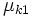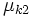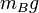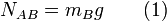# Difference between revisions of "Dragging motion for pile of blocks"

This article discusses a scenario/arrangement whose statics/dynamics/kinematics can be understood using the ideas of classical mechanics.
View other mechanics scenarios

## The two blocks scenario

Consider one block placed on top of another. The blocks are cuboidal with a horizontal surface of contact. Suppose the lower block is$A$ and the upper block is$B$, and the lower block is resting on a fixed floor. Suppose the coefficients of friction are:

•$\mu_{s1}$ is the limiting coefficient of static friction between$A$ and the floor.
•$\mu_{k1}$ is the coefficient of kinetic friction between$A$ and the floor.
•$\mu_{s2}$ is the limiting coefficient of static friction between$A$ and$B$.
•$\mu_{k2}$ is the coefficient of kinetic friction between$A$ and$B$.

We assume that there are no external forces in the vertical direction, other than the gravitational and normal force.

## Normal force and gravitational force

### On the upper block

KEY FORCE CONCEPT (ACTION-REACTION): Just because two forces are equal in magnitude and opposite in direction does not imply that they form an action-reaction pair in the sense of Newton's third law of motion. An action-reaction pair is a pair occurs between two bodies that exert forces on each other, not for a pair of forces both acting on the same body. The normal force on the upper block and$m_Bg$ (the gravitational force) are equal and opposite but do not form an action reaction pair.

If we denote by$N_{AB}$ the normal force between the blocks, then$N_{AB}$ acts upward on the upper block. The gravitational force$m_Bg$ acts downward. Since there is no net acceleration in the vertical direction, we get:$\! N_{AB} = m_Bg \qquad (1)$

## Case of force on lower block

Fill this in later

## Case of force on upper block

Fill this in later